## Photocouplers Use Light from a Light-Emitting Diode to Conduct Current through a Phototransistor

Photocouplers (also known as optocouplers) generate light by using a light-emitting diode (LED) to generate a current which is conducted through a phototransistor.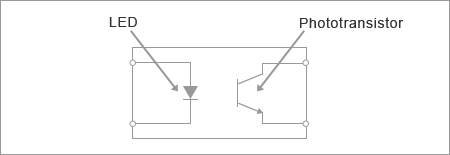Figure 1. Internal Equivalence Circuit

Here, we will describe how a general-purpose photocoupler with this basic structure is used.

Photocouplers are mainly used for the following:

• As a switching device to transfer pulse signals
• To transfer feedback error signals in analog switching regulators

The operation of photocouplers when used as switching devices is more basic, so we will start by describing this operation.

## The Transistor on the Output Side Operates as a Switch

When photocouplers are used as switching devices, the phototransistor on the output side operates simply as a switch. When a regular transistor is used as a switch, the operation differs depending on whether the load is connected to the emitter (emitter follower or common collector) or the collector (common emitter). However, in the case of general-purpose photocouplers, the base of the phototransistor is left unconnected, so the base current always flows from the collector. This means that the transistor is saturated and switches in the same way regardless of whether the load is connected to the collector or the emitter. The only difference is that the output signal polarity becomes the opposite.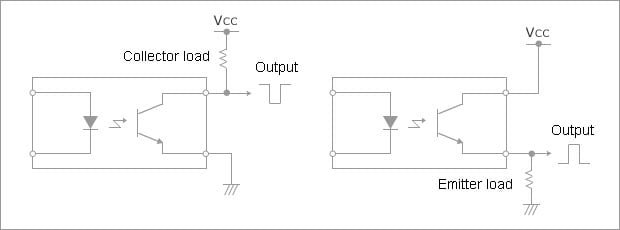## How Much is the Output Current?

Even though a photocoupler can be called a switch, its output pin cannot be connected to a heavy load such as a motor. If you look at the rated output current values for all general-purpose photocouplers, even at its highest, the current is never more than several tens of milliamperes. The circuit current capacitance should be considered simply sufficient to light the LED.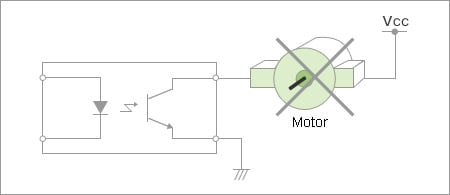Figure 3. Example of Overloaded Circuit

So how much output current actually flows in a photocoupler?

The following explanation considers the maximum output current. If you are thinking of an output current less than 2mA to 3mA, this explanation does not apply. In this case, refer to the "How Much Input Current (IF) is Required to Generate the Output Current?") section below.

1. Maximum rating input current (IF)
2. Maximum rating output current that can be generated when the input current (IF) is within the allowable range
3. Reduction in output current (IC) as characteristics degrade over time
4. Output current (IC) range in which the on-state output voltage remains below a certain value

Of these, the value of (1) is the same for both single-transistor and Darlington-transistor type photocouplers, whereas the values of (2), (3) and (4) differ depending on the transistor type. The explanation of (1) below therefore applies to all transistor types, while (2) will be explained separately for each type.

### Maximum Rating Input Current (IF)

The first question is, how much input current (IF) can be applied to a photocoupler?

The maximum rating input current (IF) is determined based on the following:

1. Current and internal power dissipation ratings
2. Degradation of characteristics over time

The strictest (smallest) value of these is specified as the maximum input current value.

(i)Determining the maximum input current value based on the current and internal power dissipation ratings.

Usually, both the current and the internal power dissipation ratings are used to determine the maximum input current value. For example, looking at the PD vs. TA graph below, you can see that the maximum rating dissipation is 75mW when the maximum operating temperature is 75 °C.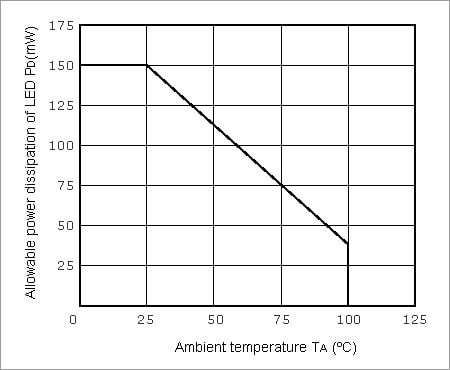Figure 4. Example for Allowable Power Dissipation of an LED (PD) vs. Ambient Temperature (TA)

At this time, if the characteristics of the LED on the input side are as shown in the graph below, the recommended forward current (IF) to achieve an internal LED power dissipation of 75mW at an ambient temperature of 75 °C is about 60mA (with a forward voltage (VF) of just over 1.2V).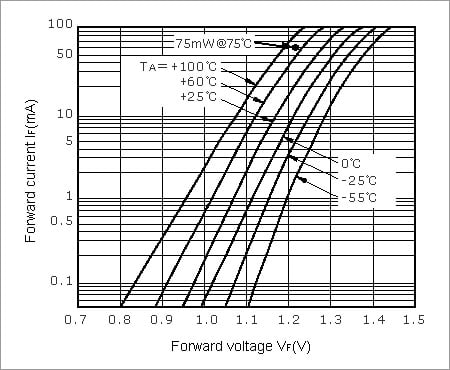Figure 5. Example LED Forward Current (IF) vs. Forward Voltage (VF)

If the current rating is larger than this value, this value becomes the input current (IF) maximum value.

(ii) Determining the maximum input current value based on the degradation of characteristics over time.

It is important to note that the light emitting effectiveness of the LED on the input side of the photocoupler degrades over time, which causes the current transfer ratio (CTR) to decrease as shown in the graph below.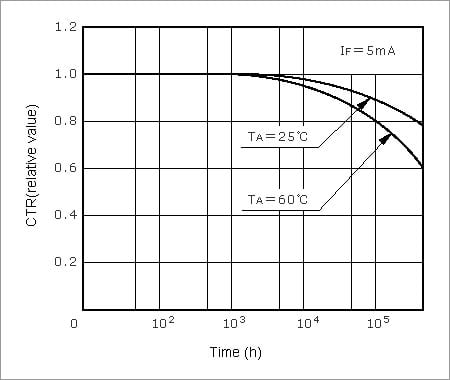Figure 6. Degradation of CTR Over Time

The input current (IF) is usually determined based on the estimated lifetime shown in the graph below.In this example, if the photocoupler is used for 100,000 hours in a location where the maximum temperature is 50 °C, the maximum input current (IF) that can be applied is 20mA. This value is the strictest value calculated thus far, so this value becomes the input current (IF) maximum value.

We will now calculate the maximum rating output current that can be generated from this input current for a single-transistor type photocoupler and for a Darlington-transistor type photocoupler.

With a Darlington transistor, IC rises suddenly as VCE approaches 1V, as shown in the graph above. If you attempt to set VCE to less than 1V in the same way as with a single transistor, an output current might not be generated at all. It is therefore generally assumed that the kind of low output voltage obtained by using a single transistor cannot be obtained when using a Darlington transistor, so VCE is set to 1.5V or less, 0.5V higher than when using a single transistor. By doing this, a collector current (IC) of 25mA can still be generated even as the photocoupler approaches the end of its life.

Because Darlington transistors have a high conductive output voltage compared with single transistors, photocouplers that use Darlington transistors are better suited to applications that require a larger output current to be generated.

### Single-Transistor Output

(i) The maximum rating output current that can be generated when the input current (IF) is within the allowable range.

As you can see in the Current Transfer Ratio (CTR) vs. Forward Current (IF) graph below, the CTR of a photocoupler usually rises quickly as the input current (IF) rises from the sampling point, but then falls again as the input current continues rising.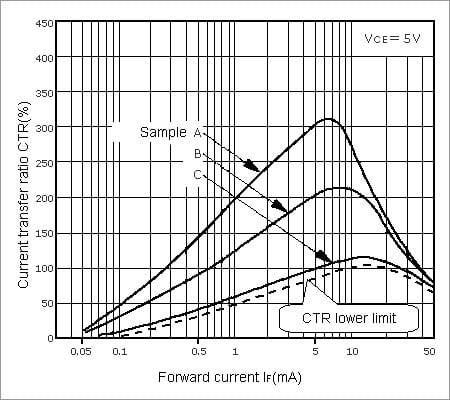Figure 8. Example Current Transfer Ratio (CTR) vs. Forward Current (IF)

The photocoupler characteristics can vary within the specification range, so a curve has been drawn in this graph that estimates the lower limit of the CTR value. If we assume a CTR of 80% when the input current (IF) is 5mA, the CTR lower limit can be estimated as shown by the dotted line in this graph. Looking at this line, it can be calculated that if an input current (IF) of 20mA (determined previously) is applied, an output current of 20mA will be generated, because the CTR when the input current (IF) is 20mA is 100%. The Maximum rating output current that can be generated is therefore 20mA. Note, however, that the following factor must also be taken into consideration when using a photocoupler:

(ii) Reduction in output current (IC) as characteristics degrade over time

The first thing that must be considered is that the above CTR value is an initial value. If the photocoupler is used for the length of time described in (ii) Determining the maximum input current value based on the degradation of characteristics over time in "(1) Maximum rating input current (IF)" above, the CTR will eventually fall to half that value.

The output current (IC) that is generated will therefore fall to 10mA, half its initial value.

(iii) Output current (IC) range in which the on-state output voltage remains below a certain value

Looking at the CTR characteristics curves in the graph above, you can see that these values apply when VCE = 5V; but is VCE = 5V actually appropriate?

This discussion of photocouplers is based on the use of the output-side transistor as a switch, so VCE must naturally be as small as possible.

Due to constraints such as the input level specifications of the logic circuits, VCE is usually set to less than 1V.

The value of the generated output current (IC) is determined based on the relationship between the collector current (IC) and the collector-emitter voltage (VCE), as shown in the graph below.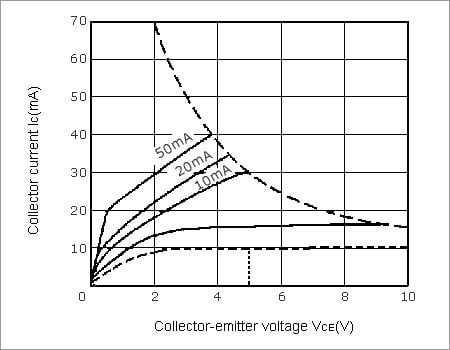Figure 9. Example Collector Current (IC) vs. Collector-Emitter Voltage (VCE)

If you draw a curve based on the values of IC = 10mA at VCE = 5V calculated in "(ii) Reduction in output current (IC) as characteristics degrade over time" above, it will look like the dotted line in the graph.

When VCE equals 1V on this curve, the value of IC is about 5mA. This is the Maximum rating output current that can be generated in switching applications, assuming that the photocoupler will be used until the end of its life. (This is simply an example; the actual values will differ depending on the product.)

If a photocoupler is used in an application in which the output current generated is larger than this, it might cause problems such as the output current not flowing at all at the start (the output signal is too small) or the product's characteristics degrading sooner than expected.

The output current that is actually allowed to be generated is therefore usually quite a lot smaller than the maximum rating.

### Darlington-Transistor Output

(i) The maximum rating output current that can be generated when the input current (IF) is within the allowable range.

A Darlington transistor has the advantage of having a large CTR.

For example, if the CTR lower limit when IF = 20mA is not 100% but 300%, an output current (IC) of 60mA can be generated at VCE = 5V.

(ii) Reduction in output current (IC) as characteristics degrade over time.

Considering the degradation of characteristics over time in the same way as a single-type transistor, IC becomes 30mA at VCE = 5V.

(iii) Output current (IC) range in which the on-state output voltage remains below a certain value.

The curve of IF = 1mA in the graph below showing the Darlington transistor collector current (IC) vs. collector-emitter voltage (VCE) approximates the values of IC = 30mA at VCE = 5V.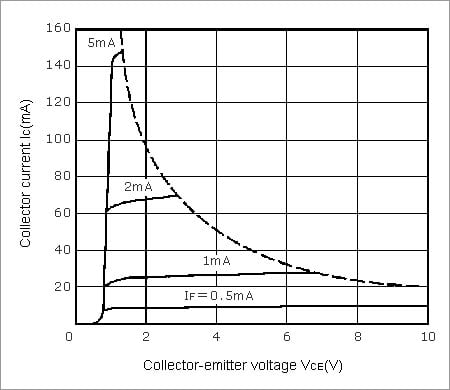Figure 10. Example Darlington Collector Current (IC) vs. Collector-Emitter Voltage (VCE)

With a Darlington transistor, however, IC rises suddenly as VCE approaches 1V, as shown in the graph above. If you attempt to set VCE to less than 1V in the same way as with a single transistor, therefore, an output current might not be generated at all.

It is therefore generally assumed that the kind of low output voltage obtained by using a single transistor cannot be obtained when using a Darlington transistor, so VCE is set to 1.5V or less, 0.5V higher than when using a single transistor.

By doing this, a collector current (IC) of 25mA can still be generated even as the photocoupler approaches the end of its life.

Because Darlington transistors have a high conductive output voltage compared with single transistors, photocouplers that use Darlington transistors are better suited to applications that require a larger output current to be generated.

Once you know the maximum output current (IC) that can be generated, the load circuit can be designed so that the output current remains below the maximum value.

Let's look at the allowable range of load resistance (RL) when using a single transistor with IC = 5mA at VCE = 1V in a circuit configuration like the one shown below, based on the results thus far.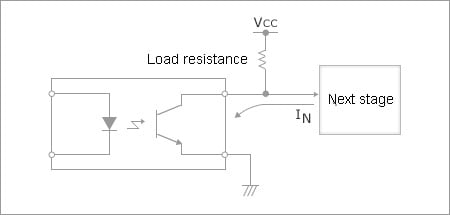Figure 11. General Circuit Configuration Example

Assuming that the current flowing into the collector from the circuit in the next stage (IN) is 1mA, if the power supply voltage (VCC) is 5V, the lower limit of the load resistance (RL) can be calculated as follows:

Equation (1)
RL>(VCC-VCE)/(IC-IN) = (5V-1V)/(5mA-1mA) = 1kΩ

If the photocoupler has a high voltage tolerance, it can be used with a high power supply voltage. For example, if a power source of 50V is used (VCC = 50V), the load resistance lower limit will be 13kΩ.

The voltage generated by the load resistor (RL) is usually set to one tenth or less of the power supply voltage (VCC) while the photocoupler is in the shutoff state and the collector dark current (ICEO) is flowing.

This dark current requires care.

Looking at the datasheet, the dark current maximum specification is 0.1µA, which seems quite small.

However, this value applies when the ambient temperature is 25 °C. The dark current (Ileak) is roughly proportional to the collector-emitter voltage (VCE), and becomes 10 times higher with every 25-degree rise in temperature.

Equation (2)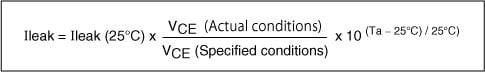For example, the maximum value of the dark current (Ileak) is 0.1µA at TA = 25 °C and VCE = 50V, but if the conditions are TA = 75 °C and VCE = 5V, the dark current decreases to one-tenth its previous value because the collector-emitter voltage is 10 times smaller, but then becomes one hundred times larger due to the rise in temperature, resulting in a 10-fold increase to a value of 1µA.

Ileak = 0.1µA x 0.1 x 102 = 0.1µA x 10 = 1µA

The load resistance required to generate a voltage that is one-tenth the value of VCE (that is, 0.5V or less) when the dark current is 1µA is 500kΩ. Based on this, if VCC = 5V, the load resistance must be 1kΩ < RL < 500kΩ.

However, this range cannot be guaranteed safe in all cases.

If the load resistance is unnecessarily high, the following problems might occur:

• The output signal level might be affected by load fluctuations.
• Noise from peripheral circuits might enter.
• The operating speed might drop.

The upper limit of the load resistance should therefore be kept to within five times the lower limit value, which when applied to the example above results in an upper limit of 5kΩ when the power supply voltage (VCC) is 5V.

## How Much Input Current (IF) is Required to Generate the Output Current?

For example, if an output current (IC) of only 2mA is required rather than 5mA, how much input current (IF) is required to generate this?

Working backwards and calculating first from the perspective of photocoupler lifetime, you can see that the initial output current generated must be twice the eventual value; that is, 4mA.

If you draw a curve for IC = 4mA at VCE = 1V on the collector current (IC) vs. collector-emitter voltage (VCE) graph shown at the beginning, it will look like the dotted line in the graph below.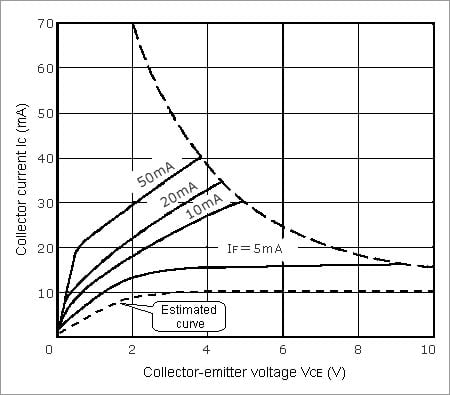Figure 12. Example Collector Current (IC) vs. Collector-Emitter Voltage (VCE)

From this graph, you can see that IC is about 10mA when VCE is 5V.

Looking at the dotted line curve in the graph above indicating the current transfer ratio (CTR) vs. forward current (IF), you can see that the CTR is approximately 100% when IF = 10mA, so if an input current of 10mA is applied, the above output current, that is an initial value of 4mA at VCE = 1V and an end-of-life value of 2mA at VCE = 1V, can be generated.

Note, however, that the input current here is estimated assuming the worst case scenario of variations in CTR and degradation over time, so if a photocoupler with a large initial CTR is used, and particularly during the early period of use, the input current might be considerably larger than the required value.

In this case, the operating speed tends to be lower than the speed that could be expected based on the specifications.

It is therefore important to check carefully and choose a photocoupler that allows you to achieve the desired operating speed.

It might also be effective to limit the range of variation by specifying a certain CTR rank.

## Operating a Photocoupler in the Active Region in Analog Applications

In analog applications, photocouplers are typically used in the feedback control loops of switching regulators in which the primary and secondary domains are isolated.

Photocouplers are used as shown in the figure below to solve the problem of how to feed back direct current while isolating the primary and secondary domains.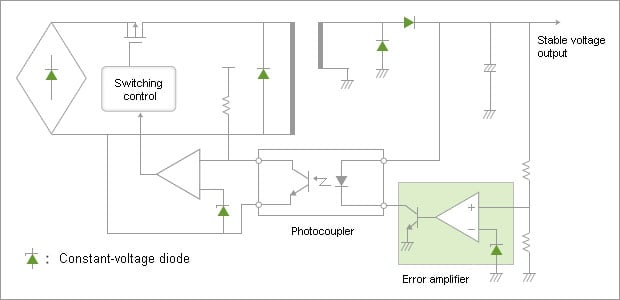Figure 13. Example of Using a Photocoupler in a Switching Regulator

When using a photocoupler in a switching regulator, the photocoupler's input is the power supply's output and vice versa.

The error amplifier, which is used to compare the output voltage of the power supply with the reference voltage (that is, determine the potential difference), is in the power supply's secondary circuit (output side). The amount of light that is applied to the photocoupler's LED differs depending on this potential difference.

The collector current of the photocoupler also differs depending on the amount of light emitted by the LED.

These factors cause a change in the phototransistor's collector-emitter voltage (VCE), which leads to an increase or decrease in the input current of the power supply, and consequently an increase or decrease in the power supply's output voltage.

At this time, as when the photocoupler is being used as a switching device, the phototransistor's load resistor can be connected to either the collector or the emitter (either is fine in terms of the operation of the photocoupler). The load resistor connection in this case is determined according to the overall circuit configuration.

However, unlike in a switching operation, when the photocoupler is being used in an analog circuit, the circuit is configured so that the phototransistor operates in a region of VCE >1V (the active region).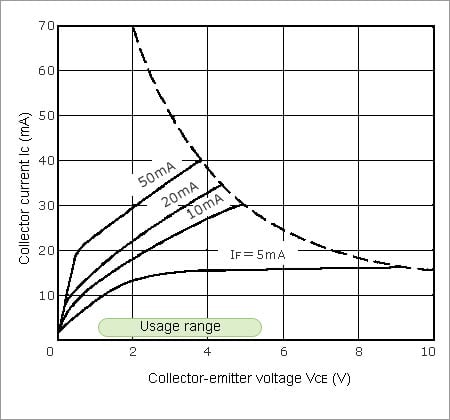Figure 14. Usage Range of a Photocoupler When Used in the Feedback Control Loop of a Switching Regulator

Also, because photocouplers tend to have very large variations in CTR, the input current control gain of the error amplifier and power supply need to be set to very large values to cope with these CTR variations.

This is the same as using amplifier circuits in audio amplifiers or operational amplifiers that have gains 100 to 1000 times higher than the gain that is actually required. These high gains are then lowered by using a feedback circuit in order to reduce signal variation and distortion.

However, one problem with using this kind of high-gain feedback controller is that it can cause parasitic resonance and other types of operational instabilities, requiring the insertion of a phase adjuster to secure a sufficient phase margin and stabilize the circuit's operation.